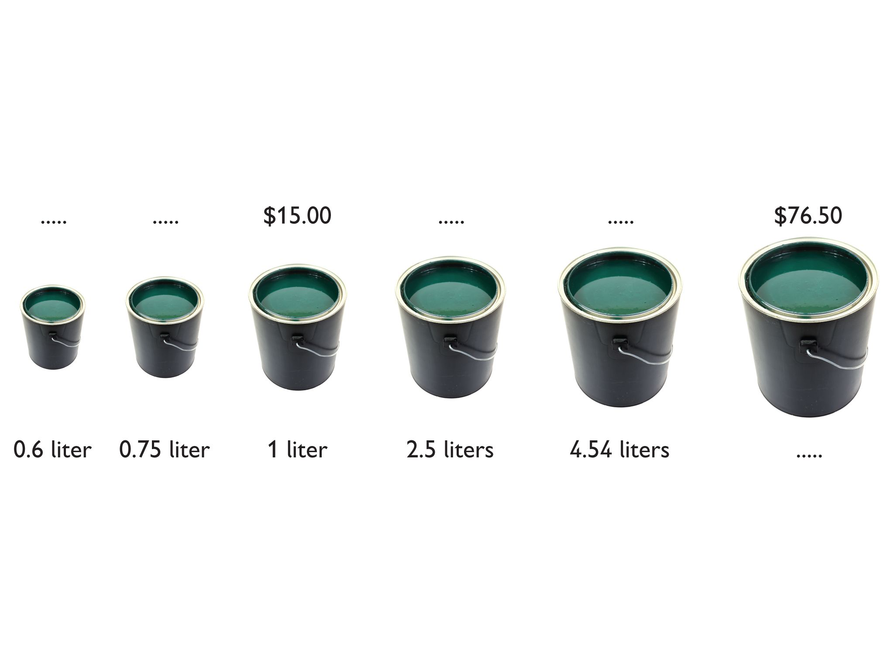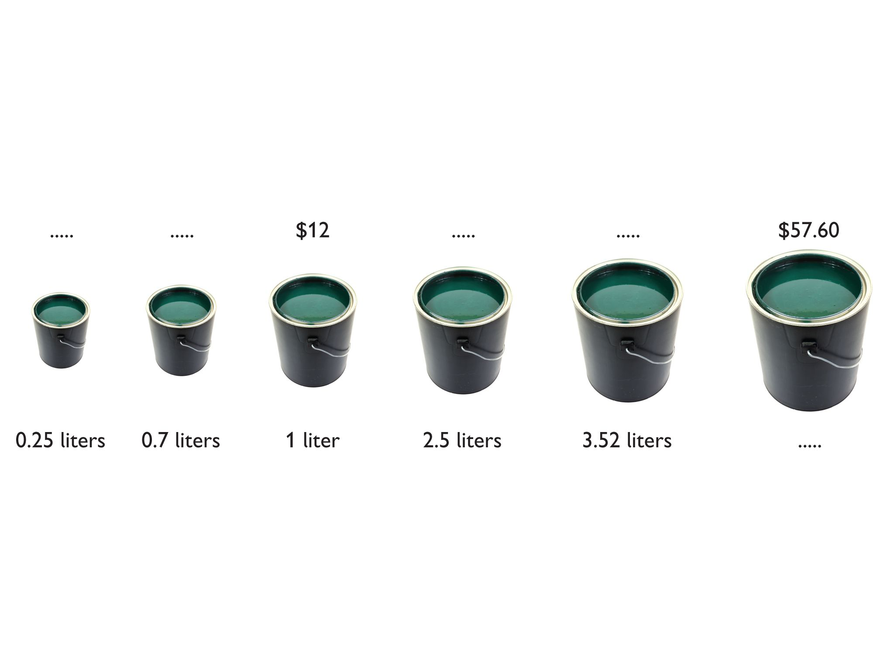Subject:
Mathematics
Material Type:
Lesson Plan
Level:
Middle School
7
Provider:
Pearson
Tags:
• Problem Solving
Language:
English
Media Formats:
Text/HTML

# Solution Strategies## Overview

Students have an opportunity to review their own work on the Self Check in the previous lesson, consider feedback that addresses specific aspects of their work, examine a different approach to the problem from the Self Check, and then use what they learned to solve a closely related problem.

# Key Concepts

Students reflect on their work, review and critique student work on the same problem, and then apply their learning to solve a similar problem.

# Goals and Learning Objectives

• Use teacher comments to refine their solution strategies for a proportional relationship problem
• Deepen their understanding of proportional relationships.
• Synthesize and connect strategies for representing and investigating proportional relationships.
• Critique given student work involving proportional relationships.
• Apply deepened understanding of proportional relationships to a new problem situation.

# Lesson Guide

Return students’ solutions to the Self Check task. If you have not added questions to individual pieces of work, write your list of questions on the board now. Students can then select questions appropriate to their own work.

Give students a few minutes to read over the feedback.

SWD: Make sure that all students have access to and can comprehend the information in the rubric so that they can accurately interpret your assessment of their work.

Students with disabilities may benefit from support in understanding the expectations. Allow multiple means of representing the information in the rubric (visual presentation of text, TTS, visual supports, etc.).

# Critique

Review your work on the Self Check problem and think about these questions.

• What are the two quantities that vary in relation to one another in this situation?
• Can you describe in words the relationship between the price and the amount of paint?
• Is there a constant of proportionality in this situation?
• Can you write a formula using the unit rate that would enable you to find one quantity if you knew the other quantity?# Lesson Guide

Discuss the Math Mission. Students will apply their knowledge of proportional relationships to solve a real-world problem and critique another student’s work.

## Opening

Apply your knowledge of proportional relationships to solve a real-world problem and critique another student’s work.

# Lesson Guide

Have students work in pairs on all problems.

Students examine sample student work on the same problem they did in the Self Check in the previous lesson. Karen’s sample work includes both errors and good strategies, though her strategies are specific to each computation rather than being generalized and informed by what students should know about proportional relationships.

SWD: One way to start the discussion is to ask students what questions arose during the pre-assessment. Student-initiated inquiry promotes connection and engagement with the mathematics. Provide positive feedback, using gestures and prompting, to elicit deeper responses.

# Mathematical Practices

Mathematical Practice 2: Reason abstractly and quantitatively.

• Watch for students who use the referents in the situation to check whether or not Karen’s (or their own) solution makes sense and who then check the values abstractly.

Mathematical Practice 3: Construct viable arguments and critique the reasoning of others.

• In critiquing the given sample student solution to the Self Check task, students must understand another person’s reasoning, recognize and analyze errors in their work, and articulate ways of improving the work.

• Identify $12 for 1 liter as a unit rate for the second paint problem. # Interventions Student only partially records his or her computation strategies. • Explain in more detail how you figured out your solution. Student uses different strategies to find the missing values. • Can you think of a solution strategy that would work for all of the missing values? Student interprets the problem structure as additive rather than multiplicative. • What are the two quantities that vary in relation to one another in this situation? • Is there a constant of proportionality between the two quantities in this situation? Student divides instead of multiplying or multiplies instead of dividing. • Make an estimate of the answer based on how big or small you expect it to be. Does your answer fit your estimate? • Use words to write down how to calculate the answer and read them aloud. Do your calculations fit your words? Student correctly uses a unit rate to find the answers. • Can you write a formula using the unit rate that works for finding all the missing values? • What would you expect the graph of the relationship in this problem to look like? Identify three features you expect the graph to have. • Sketch a graph that shows the relationship. Student uses cross-multiplication incorrectly to find the answers. • Identify which values in this computation are prices and which are amounts of paint: [insert a student’s calculation using cross-multiplication]. • Can you describe, in words, the relationship between the two quantities of paint in the calculation: [insert a student’s calculation using cross-multiplication]? • Can you describe, in words, the relationship between the price and the amount of paint in this ratio: [insert one ratio from student’s work]? Student correctly uses cross-multiplication to find the answers. • Can you explain why this method works? • Can you find a simpler way of calculating the missing values that works the same way in every case? Student finds the answers correctly and efficiently. • Find at least two different methods for finding the missing values. # Answers • Missing prices:$3.00, $8.40,$30.00, \$42.24
Missing amount of paint: 4.8 liters

# Revise and Extend Your Work

Use what you learned to solve this problem.# Lesson Guide

Make explicit comparisons between the approaches to the problem that students used and Karen’s approach. Bring out the use of unit rate. Help students clarify what is proportional to what—the quantities that are varying in relation to one another—in each case. Ask students the following:

• Does Karen’s method work for calculating any of the missing amounts in the problem? What about your own method on this problem?
• Can you think of a method to calculate any amounts (including difficult ones such as 1.23)?
• What is the unit rate in Karen’s problem? How can you use this to solve the problem?
• Why is it best to use the unit rate?

SWD: During Ways of Thinking, facilitate the learning process by encouraging students to discuss multiple strategies and representations of the mathematics. Ask questions and guide discussions. Help students to compare and contrast the variety of approaches that were used.

# Mathematical Practices

Mathematical Practice 2: Reason abstractly and quantitatively.

Students are asked to link the mathematical structure of the situation to the meaning of the quantities.

Mathematical Practice 7: Look for and make use of structure.

Students are also likely to make observations that are about the structure of proportional relationships. Be sure these comments are recognized as examples of identifying and using structure in mathematics.

# Ways of Thinking: Make Connections

Take notes about your classmates’ approaches to solving the paint can problem.

## Hint:

• What is the unit rate?
• What is the constant of proportionality?
• Does Karen’s method work for calculating any of the missing amounts in the problem?
• What advice would you give Karen?
• How did you calculate the liters when you knew the price?